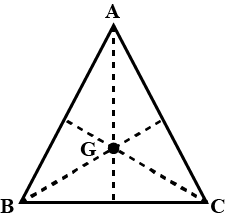Filters
Latest Questions
Physics
Gravitational force and acceleration due to gravity
What is the acceleration due to gravity on the surface of a planet that has twice the mass of the earth and half its radius?
Physics
Gravitational force and acceleration due to gravity
If the value of ‘g’ at a place is decreased by $2\%$ . The barometric height of the mercury
A.Increases by $2\%$
B.Decreases by $2\%$
C.Remains unchanged
D.Sometimes increases and sometimes decreases
Physics
Gravitational force and acceleration due to gravity
If a particle takes $t$ second less and acquires the velocity of $v$ $m/s$ more in falling through the same distance on to the planets where the acceleration due to gravity are $2g$ and $8g$ respectively. Then,
A. $4gt$
B. $5gt$
C. $2gt$
D. $16gt$
Physics
Gravitational force and acceleration due to gravity
A false balance has equal arms. An object weighs ${w_1}$ when placed in one pan and ${w_2}$ when placed in another pan. Then the weight $w$ of the object is:
A. $\sqrt {{w_1}{w_2}}$
B. $\dfrac{{{w_1} + {w_2}}}{2}$
C. $\dfrac{{{w_1}^2 + {w_2}^2}}{2} - 1$
D. $\sqrt {{w_1}^2 + {w_2}^2}$
Physics
Gravitational force and acceleration due to gravity
A stone resting on the ground has a gravitational force of 20 N acting on it. What is the weight of the stone? What is its mass? ($g=10\text{ }m{{s}^{-2}}$).
Physics
Gravitational force and acceleration due to gravity
What must be the angular velocity of rotation of the earth so that the effective acceleration due to gravity at the equator is zero? The radius of the earth = $64 \times {10^4}\,m$ .
A. $3.3 \times {10^{ - 3}}\,rad\,{s^{ - 1}}$
B. $3.5 \times {10^{ - 3}}\,rad\,{s^{ - 1}}$
C. $3.7 \times {10^{ - 3}}\,rad\,{s^{ - 1}}$
D. $3.9 \times {10^{ - 3}}\,rad\,{s^{ - 1}}$
Physics
Gravitational force and acceleration due to gravity
What are the differences between the Gravitational force and the Force of Gravity?
Physics
Gravitational force and acceleration due to gravity
Three equal masses of $\;m$ kg each are fixed at the vertices of an equilateral triangle $ABC$.
a. What is the force acting on a mass $2m$ placed at the centroid G of the triangle?
b. What is the force if the mass at vertex A is doubled? Take $AG = BG = CG = 1m$.Physics
Gravitational force and acceleration due to gravity
Three identical point masses, each of mass $1\,kg$ lie in the x-y plane at points $\left( {0,0} \right)$ ,$\left( {0,0.2\,m} \right)$ and $\left( {0.2\,m,0} \right)$ . The gravitational force on the mass at the origin:
A. $1.67 \times {10^{ - 9}}\left( {\widehat i + \widehat j} \right)N$
B. $3.34 \times {10^{ - 10}}\left( {\widehat i + \widehat j} \right)N$
C. $1.67 \times {10^9}\left( {\widehat i - \widehat j} \right)N$
D. $3.34 \times {10^{10}}\left( {\widehat i - \widehat j} \right)N$

Physics
Gravitational force and acceleration due to gravity
What happens to the gravitational force between two objects when the distance between them is:
(i) doubled?
(ii) halved?
Physics
Gravitational force and acceleration due to gravity
What is the dimension of the quantity$l\sqrt {\dfrac{l}{g}}$, l being the length and g is the acceleration due to gravity.

Physics
Gravitational force and acceleration due to gravity
What must be the angular velocity of rotation of so that the effective acceleration due to gravity equal to zero. The Radius of the earth $=$$64\times {{10}^{4}}m$
A. $3.3\times {{10}^{-3}}\dfrac{rad}{s}$
B. $3.5\times {{10}^{-3}}\dfrac{rad}{s}$
C. $3.7\times {{10}^{-3}}\dfrac{rad}{s}$
D. $3.9\times {{10}^{-3}}\dfrac{rad}{s}$

Prev
1
2
3
4
5